#Function Repository Resource:

# AreaBetweenCurves (5.3.0)current version: 5.3.2 »

Find the area between two plane curves

Contributed by: Wolfram|Alpha Math Team
 ResourceFunction["AreaBetweenCurves"][{f,g},{x,xmin,xmax}] finds the area of the enclosed region between the functions f(x) and g(x) over the interval xmin < x < xmax.

## Details

ResourceFunction["AreaBetweenCurves"] works with real‐valued functions over the Cartesian coordinate system.
The area between f(x) and g(x) is defined as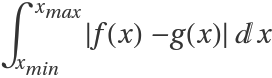.
When f(x)g(x), the area between the two curves is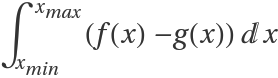.
When f(x) and g(x) only meet at x=xmin and x=xmax, the area is taken to be that of the enclosed region.
When f(x) and g(x) do not meet at x=xmin or x=xmax, the boundary of the enclosed region will contain vertical line segments joining the curves.
When f(x) and g(x) intersect for some xmin < x < xmax, the area will be that of multiple enclosed regions.
The following option can be given:
 Assumptions \$Assumptions assumptions on parameters

## Examples

### Basic Examples (1)

Find the area between two curves:

 In:=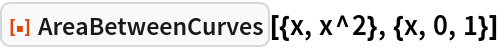Out=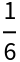In:=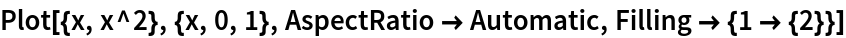Out=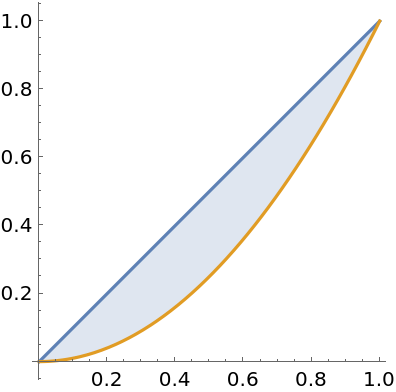### Scope (4)

Find the area of the region enclosed by two curves:

 In:=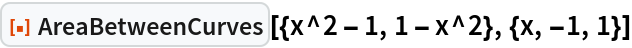Out=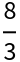In:=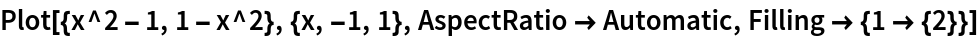Out=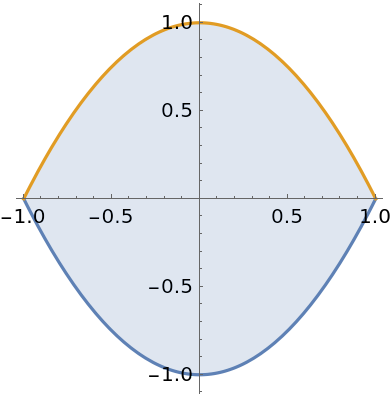Where the curves do not meet:

 In:=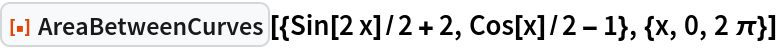Out=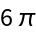In:=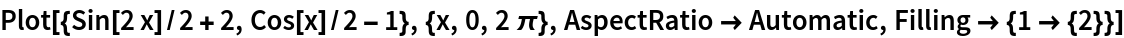Out=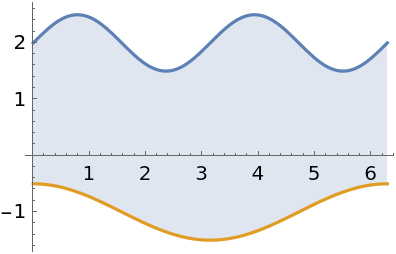With multiple enclosed regions:

 In:=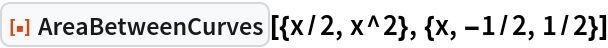Out=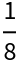In:=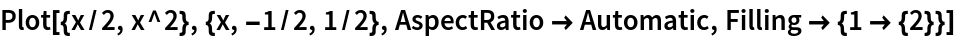Out=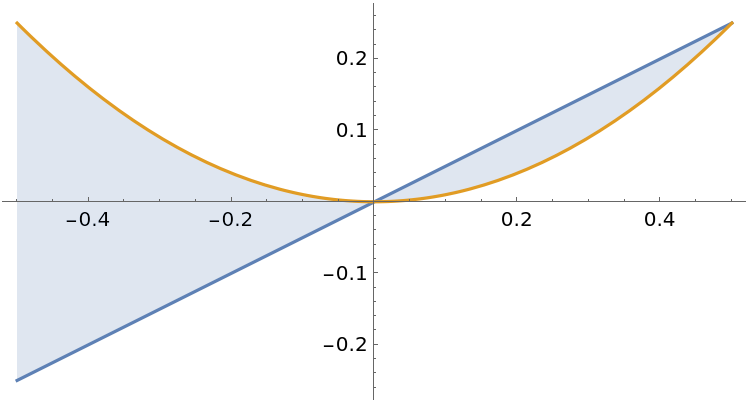Between curves containing parameters:

 In:=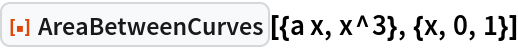Out=### Generalizations and Extensions (3)

Find the area over an unbounded interval:

 In:=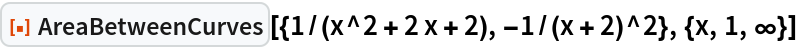Out=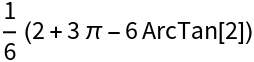In:=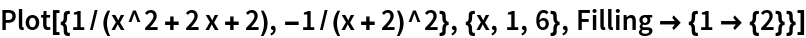Out=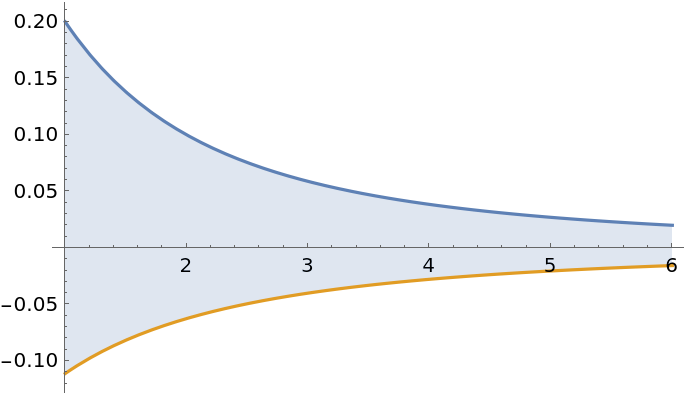Curves with discontinuities over intervals:

 In:=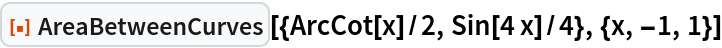Out=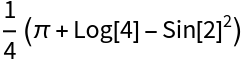In:=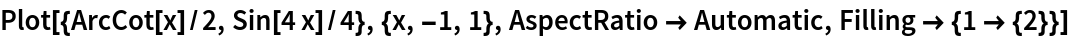Out=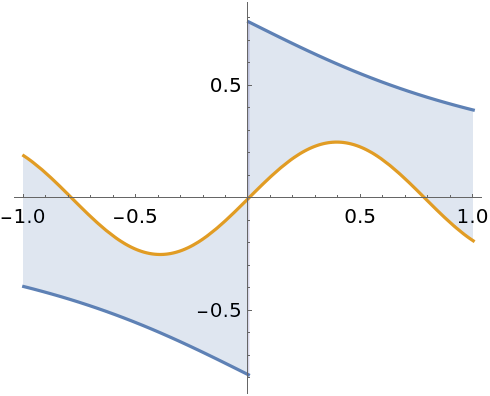With singularities:

 In:=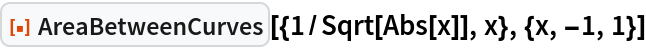Out=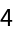In:=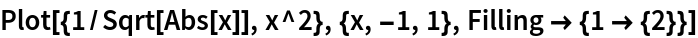Out=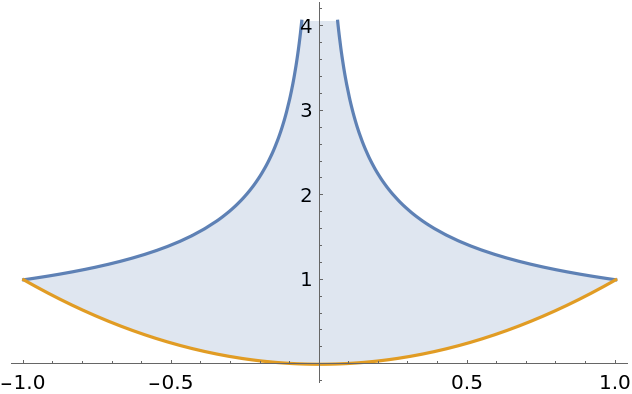### Options (2)

#### Assumptions

The result may be conditioned on parameters:

 In:=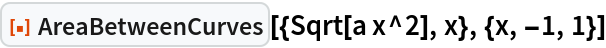Out=Make an assumption about the parameter:

 In:=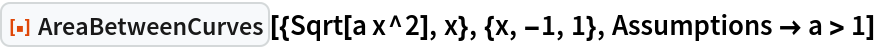Out=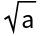### Applications (3)

Compute the area of a disk:

 In:=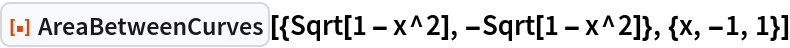Out=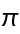In:=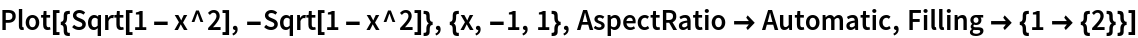Out=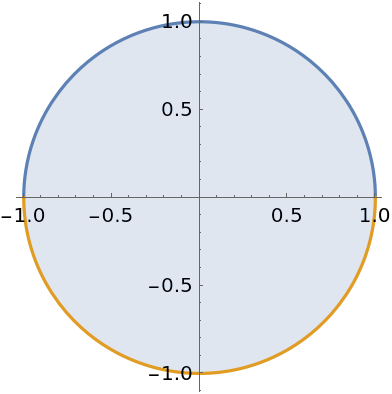Cavalieri's principle states that the area between two curves does not change when each curve is shifted by the same amount:

 In:=In:=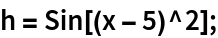In:=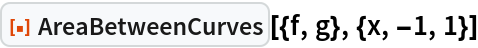Out=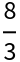In:=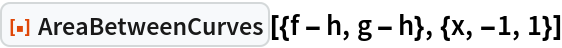Out=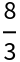In:=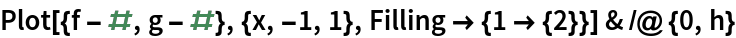Out=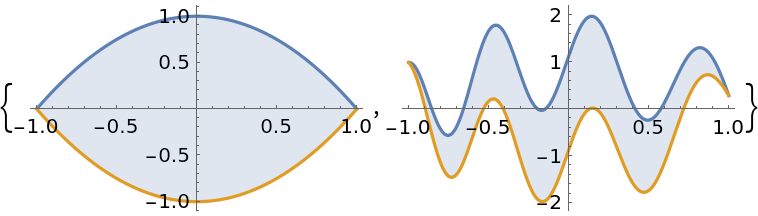The population of a region is currently growing at a rate of 35.208 ⅇ0.0083 t hundred people per year. It is thought that a large spike in employment opportunities can drop the growth rate to 24.098 ⅇ0.0071 t hundred people per year over the next five years. Find how many fewer people will be born if such a spike occurs:

 In:=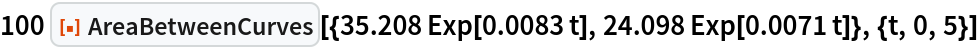Out=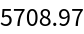### Properties and Relations (5)

Area is always non-negative:

 In:=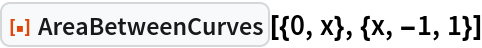Out=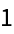The order in which the curves are specified does not matter:

 In:=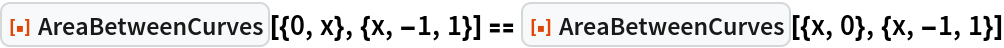Out=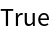Find the area of multiple enclosed regions:

 In:=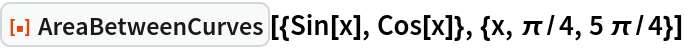Out=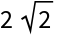Sum over each enclosed region instead:

 In:=Out=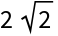In:=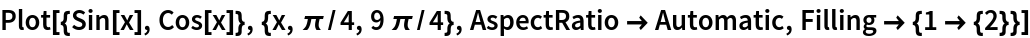Out=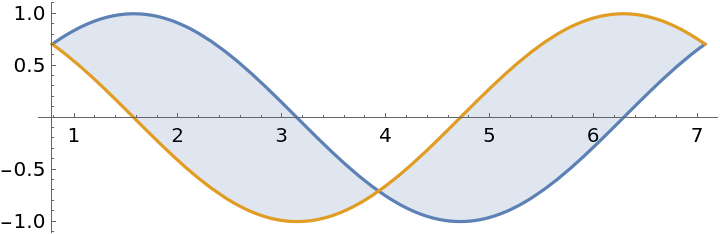The area between two curves is the integral of the absolute value of their difference:

 In:=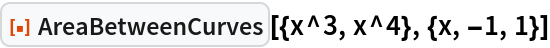Out=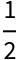In:=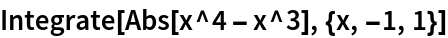Out=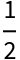### Possible Issues (2)

The integral defining the area between two curves may not converge:

 In:=Out=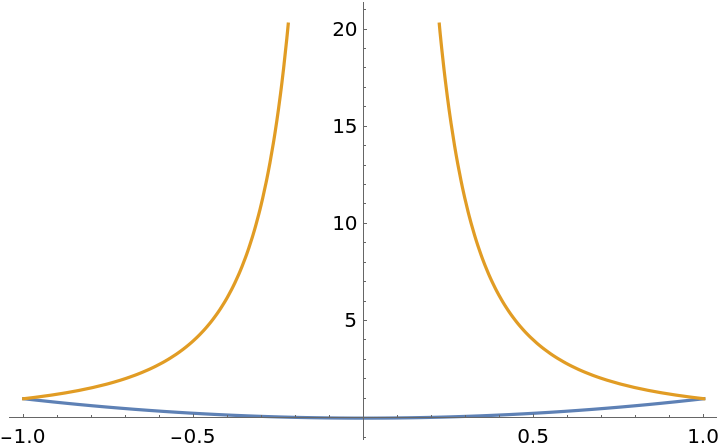In such cases, AreaBetweenCurves throws a message:

 In:=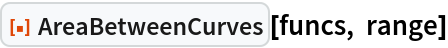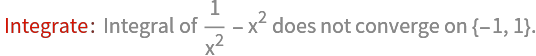Out=Functions must be real-valued over the entire range of integration. Here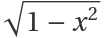is imaginary for x>1:

 In:=Out=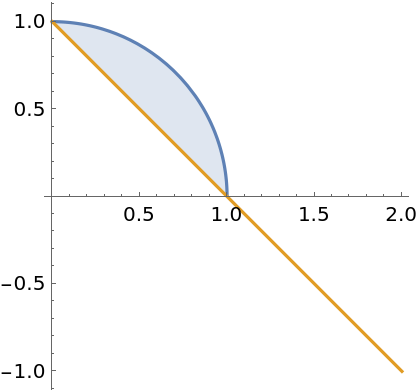AreaBetweenCurves throws a message to warn the user:

 In:=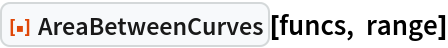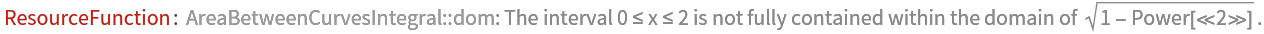Out=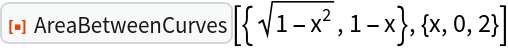Restricting the domain of integration yields a result:

 In:=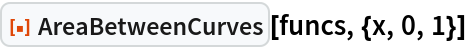Out=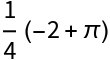## Publisher

Wolfram|Alpha Math Team

## Version History

• 5.3.2 – 22 March 2023
• 5.3.1 – 22 March 2023
• 5.3.0 – 12 May 2021